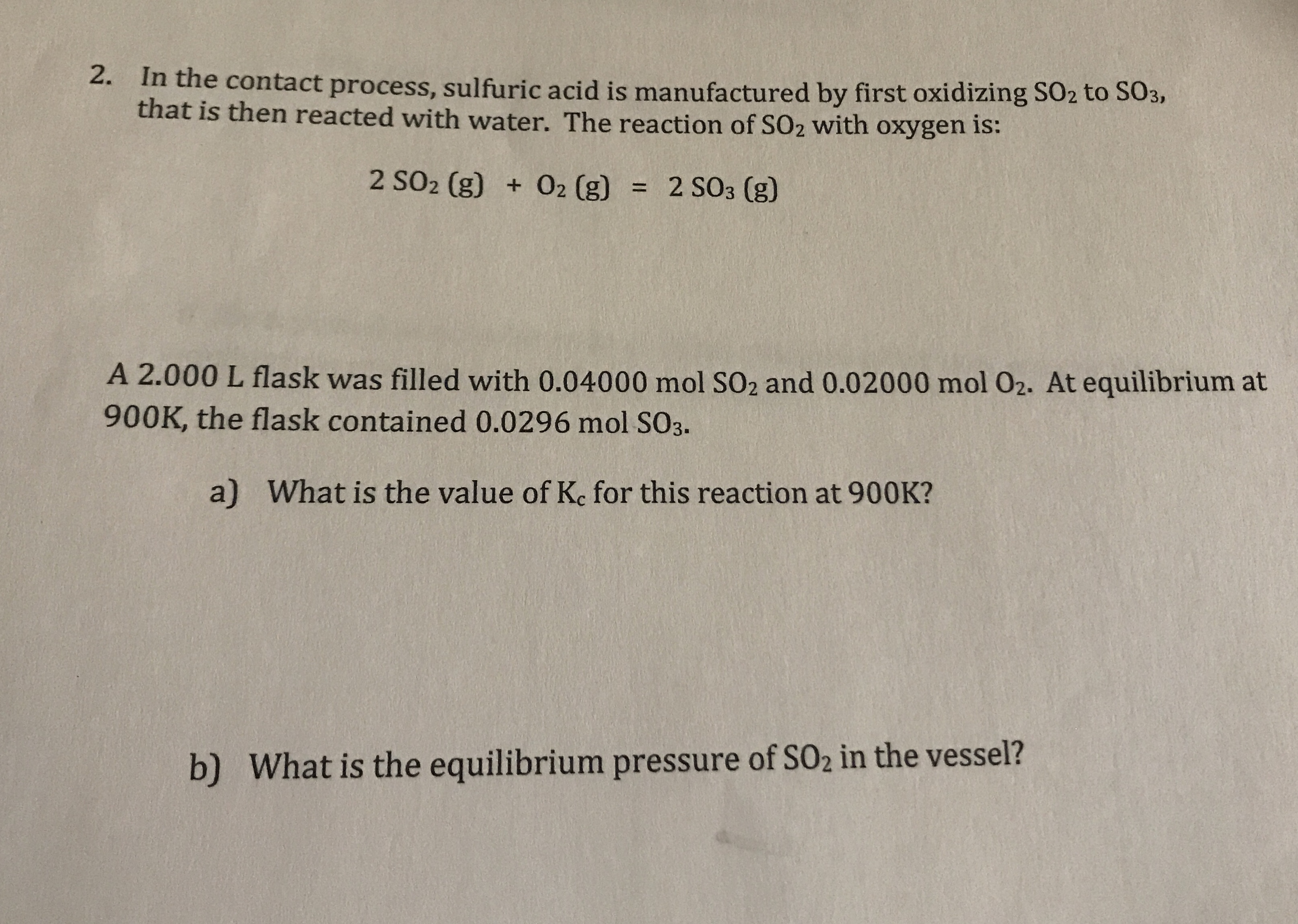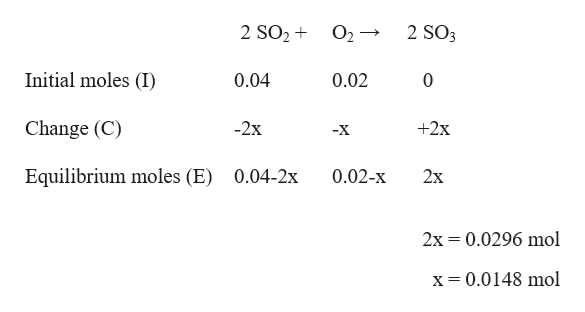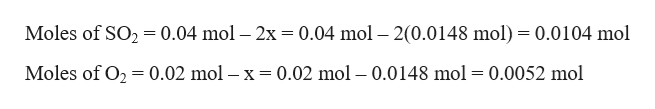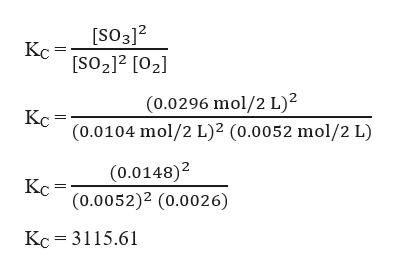# 2.In the contact process, sulfuric acid is manufactured by first oxidizing SO2 to S03,that is then reacted with water. The reaction of SO2 with oxygen is:2 SO2 (g) 02 (g)2 SO3 (g)A 2.000 L flask was filled with 0.04000 mol SO2 and 0.02000 mol 02. At equilibrium at900K, the flask contained 0.0296 mol SO3.a)What is the value of Ke for this reaction at 900K?What is the equilibrium pressure of SO2 in the vessel?b)

Question
2 viewshelp_outlineImage Transcriptionclose2. In the contact process, sulfuric acid is manufactured by first oxidizing SO2 to S03, that is then reacted with water. The reaction of SO2 with oxygen is: 2 SO2 (g) 02 (g) 2 SO3 (g) A 2.000 L flask was filled with 0.04000 mol SO2 and 0.02000 mol 02. At equilibrium at 900K, the flask contained 0.0296 mol SO3. a) What is the value of Ke for this reaction at 900K? What is the equilibrium pressure of SO2 in the vessel? b) fullscreen
check_circle

Step 1

Given,

Volume, V = 2 L

Temperature, T = 900 K

Initial moles of SO2 = 0.04 mol

Initial moles of O2 = 0.02 mol

Equilibrium moles of SO3 = 0.0296 mol

The given reaction is :

2 SO2 (g) + O2 (g) → 2 SO3 (g)

The ICE table for this reaction can be written as:help_outlineImage Transcriptionclose2 SO2 2 SO3 O2 Initial moles (I) 0.02 0.04 0 Change (C) 2x +2x -X Equilibrium moles (E) 0.02-x 0.04-2x 2х 2x 0.0296 mol x= 0.0148 mol fullscreen
Step 2

At equilibrium, moles of SO2 and O2 are:help_outlineImage TranscriptioncloseMoles of SO2 = 0.04 mol - 2x = 0.04 mol - 2(0.0148 mol) = 0.0104 mol Moles of O2 0.02 mol - x= 0.02 mol - 0.0148 mol 0.0052 mol fullscreen
Step 3

Therefore, the equilibrium constant, KC...help_outlineImage Transcriptionclose[SO312 Кс [SO2]2 [O2 (0.0296 mol/2 L)2 (0.0104 mol/2 L)2 (0.0052 mol/2 L) Кс (0.0148)2 Кс (0.0052)2 (0.0026) Kc 3115.61 fullscreen

### Want to see the full answer?

See Solution

#### Want to see this answer and more?

Solutions are written by subject experts who are available 24/7. Questions are typically answered within 1 hour.*

See Solution
*Response times may vary by subject and question.
Tagged in

### Equilibrium Concepts